Homework Help Question & Answers

# (10 pts) A solution is prepared by dissolving 1.239 grams of hydrochloric acid, HCI, in enough...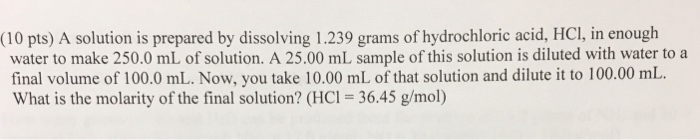(10 pts) A solution is prepared by dissolving 1.239 grams of hydrochloric acid, HCI, in enough water to make 250.0 mL of solution. A 25.00 mL sample of this solution is diluted with water to a final volume of 100.0 mL. Now, you take 10.00 mL of that solution and dilute it to 100.00 mL What is the molarity of the final solution? (HCI 36.45 g/mol)

#### Homework Answers

Answer #1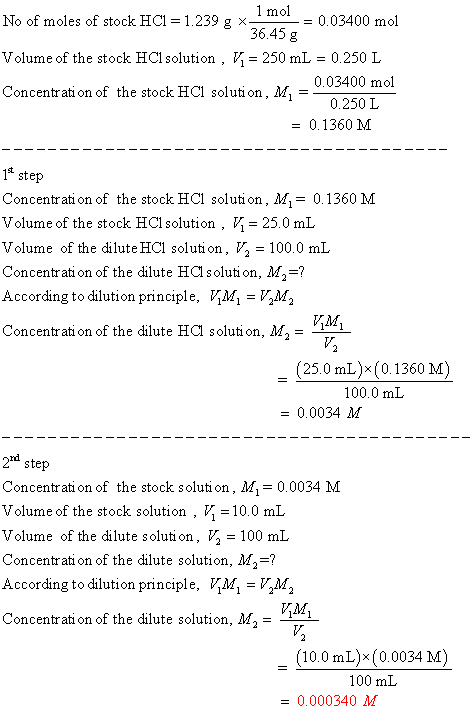Know the answer?
Your Answer:

#### Post as a guest

Your Name:

What's your source?

#### Earn Coin

Coins can be redeemed for fabulous gifts.

Not the answer you're looking for? Ask your own homework help question. Our experts will answer your question WITHIN MINUTES for Free.
Similar Homework Help Questions
• ### 2(10 points) A solution is prepared by dissolving 25.00 grams o f ba rium nitrate, Ba(NO)...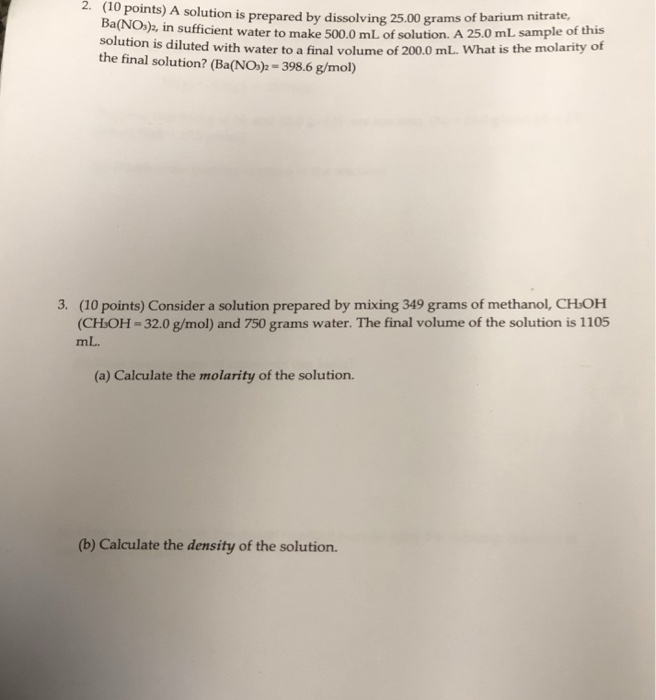2(10 points) A solution is prepared by dissolving 25.00 grams o f ba rium nitrate, Ba(NO) in sufficient water to make 500.0 mL of solution. A 25.0 mL sample of this is diluted with water to a final volume of 200.0 mL. What is the molarity of the final solution? (Ba(NO>-398.6 g/mol) 3. (10 points) Consider a solution prepared by mixing 349 grams of methanol, CHOH CHOH-320 g/mol) and 750 grams water. The final volume of the solution is 1105...

• ### 1) (a) Calculate the molarity of a solution made by dissolving 12.5 grams of Na2CrO4 on enough...

1) (a) Calculate the molarity of a solution made by dissolving 12.5 grams of Na2CrO4 on enough water to form exactly 550 ml of solution. (b) How many moles of KBr arepresent in 150 ml of a 0.275 M solution? (c) How many milliliters of 6.1 M HCI solution are needed to obtain 0.100 mol of HCI?2) (a) How many grams of solute are persent in 15.0 ml of 0.736 M K2Cr2O7 ? (b) if 14.00 g of (NH4)2SO4 is...

• ### i need help with 1-6 Carlos Chapter 9 Practice Problems t. A solution is made by dissolving 50.0 grams of HCI in enough water to give 2500 ml. of selutien. a) What is the molarity of the solution?...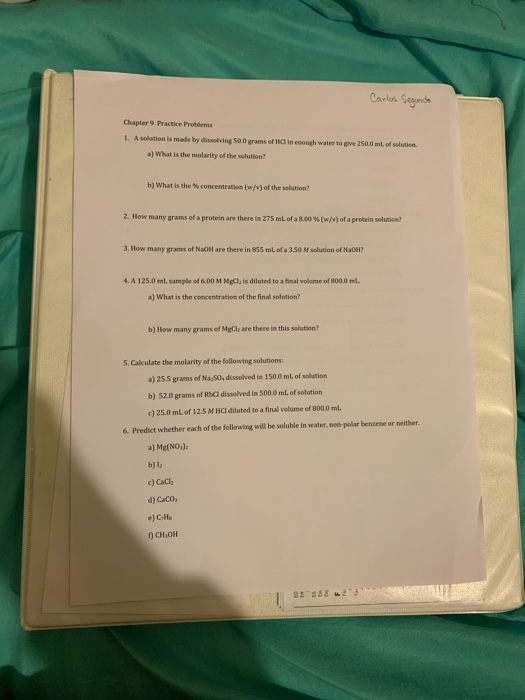i need help with 1-6 Carlos Chapter 9 Practice Problems t. A solution is made by dissolving 50.0 grams of HCI in enough water to give 2500 ml. of selutien. a) What is the molarity of the solution? b) what is the % concentration (w/v) of the solution? 2. How many grams of a protein are there in 275 mL of a 0.00%(wmor a protein soluton? 3. How many grams of NaoH are there in 855 mL of a 350...

• ### 8. A solution is made by dissolving 12.3 grams of calcium nitrate in enough water to...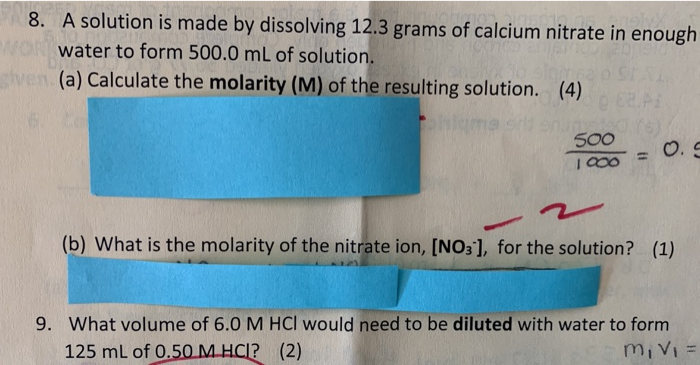8. A solution is made by dissolving 12.3 grams of calcium nitrate in enough water to form 500.0 mL of solution. sve (a) Calculate the molarity (M) of the resulting solution. (4) (b) What is the molarity of the nitrate ion, [NO3-), for the solution? (1) 9. What volume of 6.0 M HCl would need to be diluted with water to form 125 mL of 0.50 M HCI? (2) mivi

• ### Question 25 of 35 > What is molarity of A1+ ions in a solution prepared by...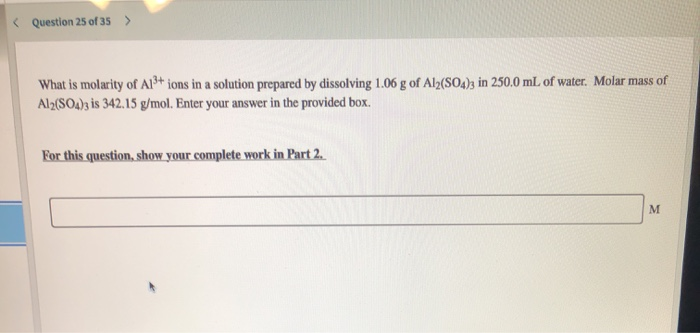Question 25 of 35 > What is molarity of A1+ ions in a solution prepared by dissolving 1.06 g of Al2(SO4)3 in 250,0 mL of water. Molar mass of Al2(SO4)3 is 342.15 g/mol. Enter your answer in the provided box. For this question, show your complete work in Part 2 Question 24 of 35 > An aqueous potassium iodate stock solution is made by dissolving 5.86 mol KIO, in sufficient water for the final volume of the solution to be...

• ### Standard solutions of calcium ion used to test for water hardness are prepared by dissolving pure...

Standard solutions of calcium ion used to test for water hardness are prepared by dissolving pure calcium carbonate, CaCO3, in dilute hydrochloric acid. A 1.212 g sample of CaCO3 is placed in a 200.0 mL volumetric flask and dissolved in HCI. Then the solution is diluted to the calibration mark of the volumetric flask. Calculate the resulting molarity of calcium ion.

• ### A solution is prepared by dissolving 17.75 g sulfuric acid, H2SO4, in enough water make 100.0...

A solution is prepared by dissolving 17.75 g sulfuric acid, H2SO4, in enough water make 100.0 mL. What is the molarity? A) 1.940 M H2SO\$ B) 0.1810 M H2SO4 C) 1.810 M H2SO4 D) 0.1775 M H2SO4

• ### A stock solution of Na_2S_2O_3 is prepared by adding 20.40 grams of solid Na_2S_2 O_3 to...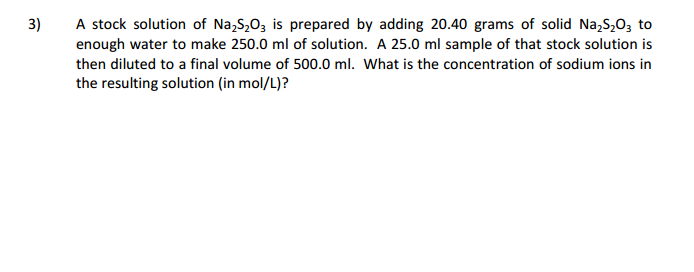A stock solution of Na_2S_2O_3 is prepared by adding 20.40 grams of solid Na_2S_2 O_3 to enough water to make 250.0 ml of solution. A 25.0 ml sample of that stock solution is then diluted to a final volume of 500.0 ml. What is the concentration of sodium ions in the resulting solution (in mol/L)?

• ### 3. A solution is prepared by dissolving 12.5 g of glucose (CHarO%) in 100.0 g of...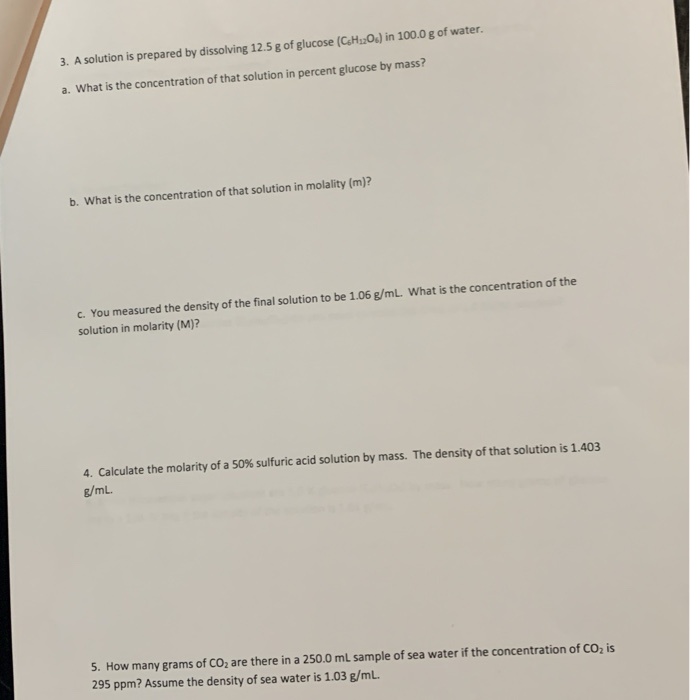3. A solution is prepared by dissolving 12.5 g of glucose (CHarO%) in 100.0 g of water. a. What is the concentration of that solution in percent glucose by mass? b. What is the concentration of that solution in mola lity (m)? c. You measured the density of the final solution to be 1.06 g/m L. What is the concentration of the solution in molarity (M)? 4. Calculate the molarity of a 50% sulfuric acid solution by mass. The density...

• ### A solution is prepared by dissolving 47.8 grams of glucose (180.16 g/mol) in 0.159 kilograms of water. The final volume of the solution is 441 mL . Calculate the molarity of the solution.

A solution is prepared by dissolving 47.8 grams of glucose (180.16 g/mol) in 0.159 kilograms of water. The final volume of the solution is 441 mL . Calculate the molarity of the solution.

Free Homework App

Scan Your Homework
to Get Instant Free Answers
Need Online Homework Help?

Get Answers For Free
Most questions answered within 3 hours.